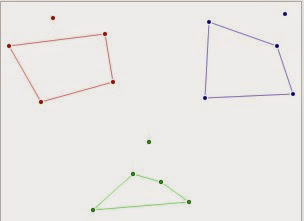Thursday, 28 August 2014

Not The End for the Happy Ending Couple*Wik

On 28 August 2005, Esther Klein and her husband George passed away within an hour of each other. An unusual event made even more interesting by a beautiful mathematical problem, that linked them together, and spawned the mathematical areas called Ramsey Theory, and Combinatorial Geometry.

In 1933 a group of mostly male students met regularly in Budapest to discuss mathematics. At one such meeting, one of (perhaps the only??) women present, Esther Klein, asked a simple geometric question: Is it possible to place five points on a plane so that no four of them form a convex quadrilateral? None of the student's present could answer her challenge; a fact made more impressive in that one of the students was Paul Erdos, one of the most prolific problem solvers in mathematics history.  Another student present was George Szekeres, another prolific mathematician working in combinatorial mathematics and a prominent player in the problem Esther submitted.

Esther then went on to illustrate her proof. Today the problem and it's generalization is regarded as one of the foundational works in the field of combinatorial geometry.

Within four years, Esther and George were married, and Paul Erdos dubbed the problem the Happy Ending Problem as he felt it was the start of their relationship.

The common proof used today for the problem is to divide it into simple cases. It is assumed that the points are in general position, that is, no three are collinear. Pick three of the points to form a triangle. If any point(s) is outside the triangle, then a convex quadrilateral can be drawn using four points. For the case when the two other points are inside the triangle, the segment containing these two points and one side of the triangle can be united into a convex quadrilateral. Also see the nice illustration at Theorem of the Day.

Erdos and George Szekeres generalized the problem to the theorem: For any positive integer N, any sufficiently large finite set of points in the plane in general position has a subset of N points that form the vertices of a convex polygon.
But HOW BIG was a "sufficiently large finite set of points" for a given convex n-gon? For a triangle, three points was all that was necessary. For a quadrilateral, Esther had shown that five points would suffice. Erdos and George predicted that for a pentagon, it would require nine points, but the complete proof was not published until 1970. Shortly after the death of George and Esther Szekeres, the solution for a hexagon was published in the ANZIAM Journal. The paper, Computer solution to the 17-point Erdös-Szekeres problem by George Szekeres(deceased)and
Lindsay Peters, showed that for a convex hull of six sides, the required number would be 17 points. (A challenging problem for students would be to create 16 points in general position so that no six formed a convex hexagon.)
Beyond that.... we just don't know. It must be a finite number, and we know from another Erdos-Sekeres proof that for an n-gon, the number of points is greater than or equal to 1 + 2(n-2).

Paul Erdos used to talk about "God's Book." A list of all the best solutions to every mathematical problem. Maybe they got a peak after they left this plane. And Happily, the generalized Happy Ending problem has not ended for us still here. Care to try for a convex heptagon.